Question

# Please answer correctly I'll give best rating if you do correct one Electron Geomctry Name Choices:...

Please answer correctly I'll give best rating if you do correct one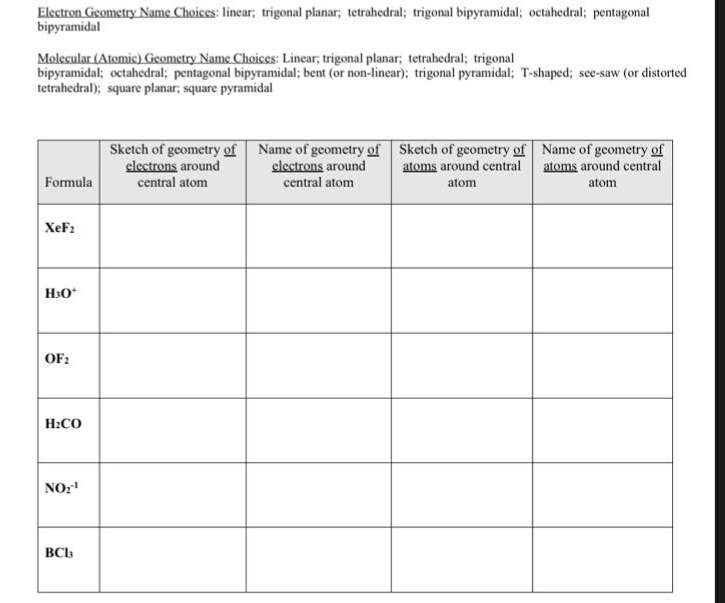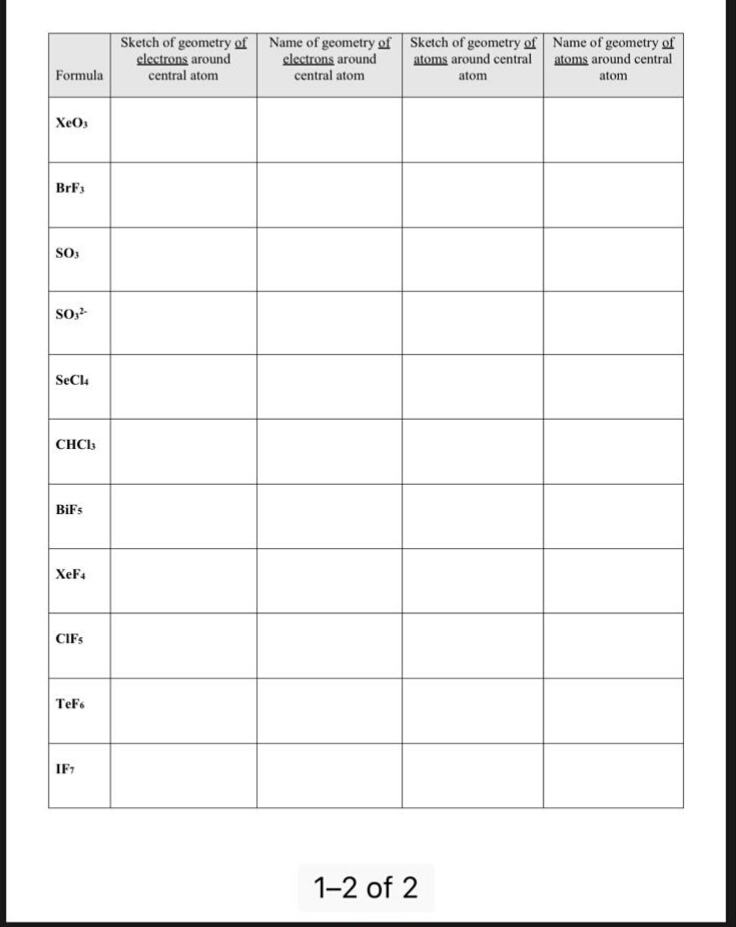Electron Geomctry Name Choices: linear; trigonal planar; tetrahedral; trigonal bipyramidal; octahedral; pentagonal bipyramidal Molecular (Atomic) Geometry Name Choices: Linear, trigonal planar; tetrahedral; trigonal bipyramidal; octahedral; pentagonal bipyramidal; bent (or non-linear); trigonal pyramidal: T-shaped; see-saw (or distorted tetrahedral); square planar, square pyramidal Sketch of geometry of Name of geometry of Sketch of geometry of Name of geometry of clectrons around central atom clectrons around atoms around central atoms around central atom Formula central atom atom XeF2 H30 OF2 H2CO BC13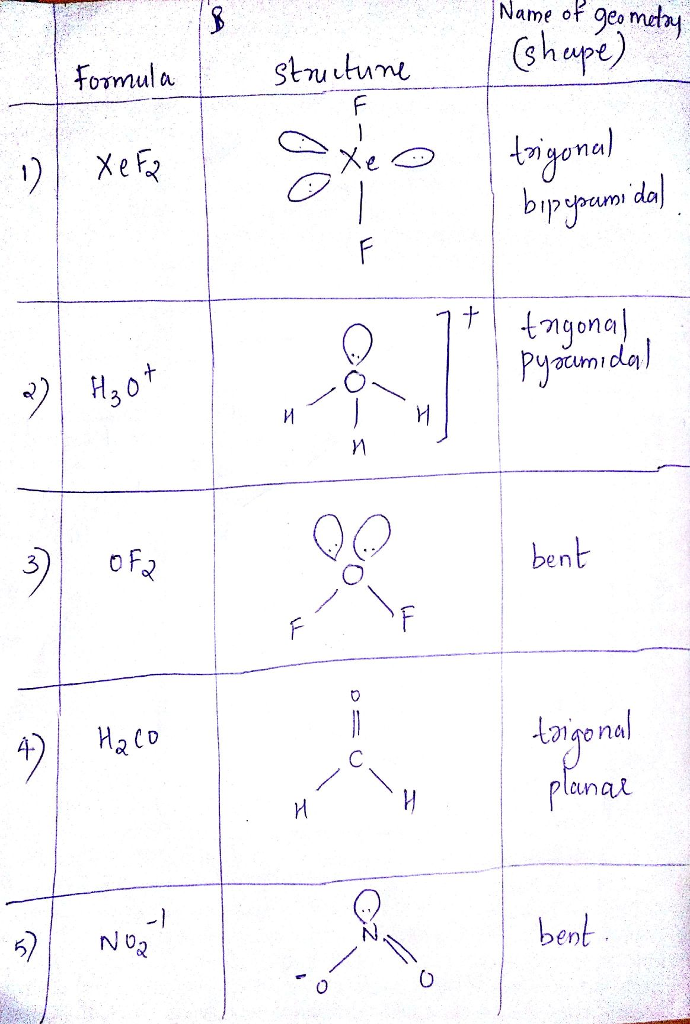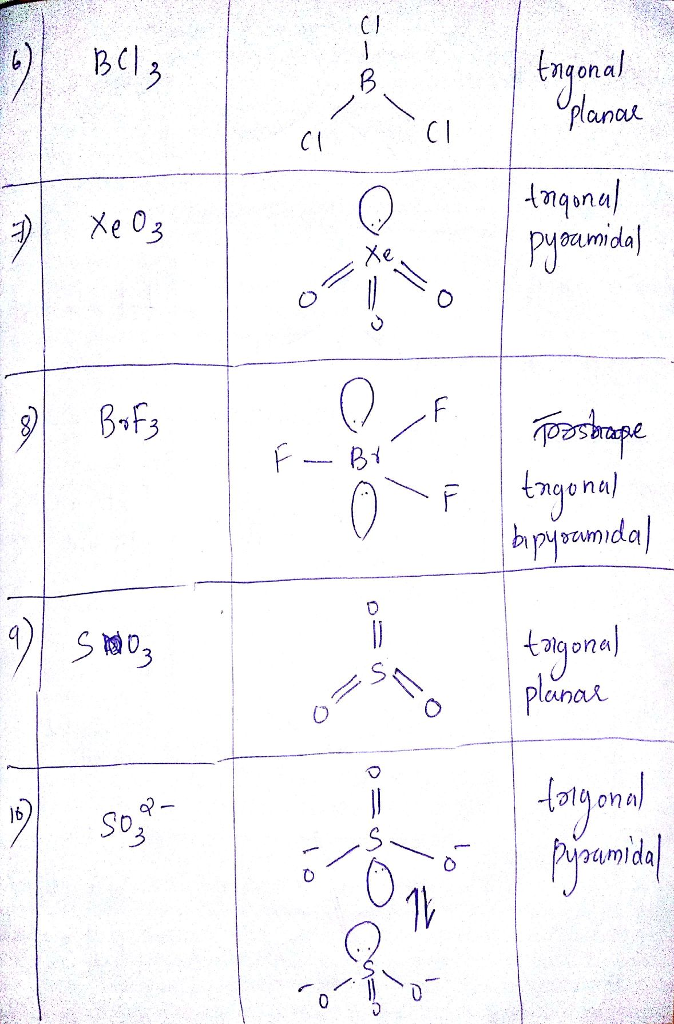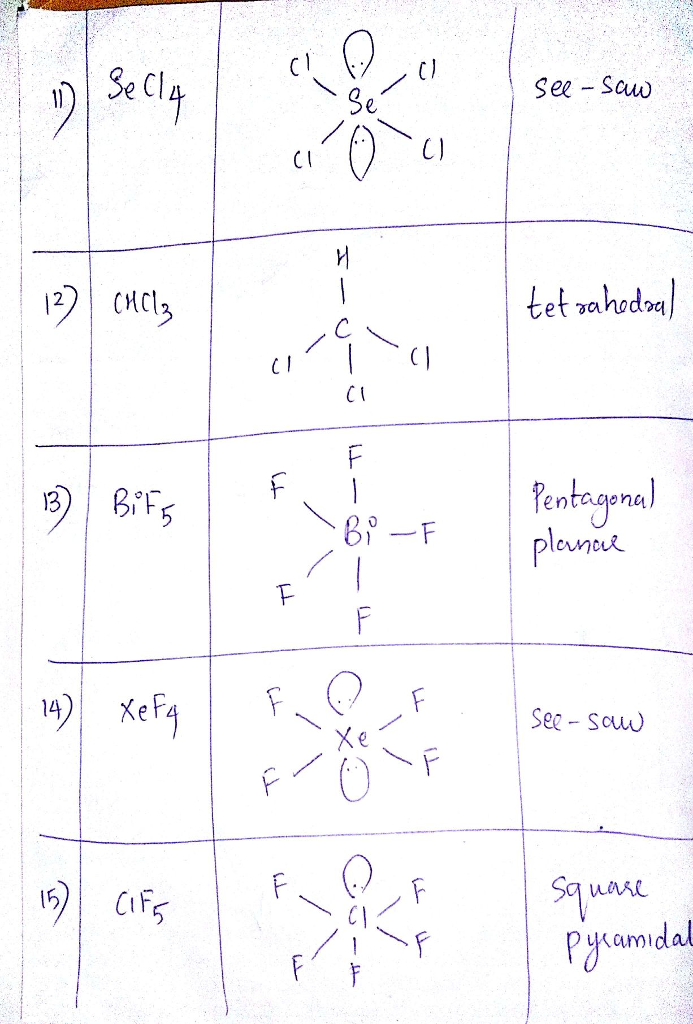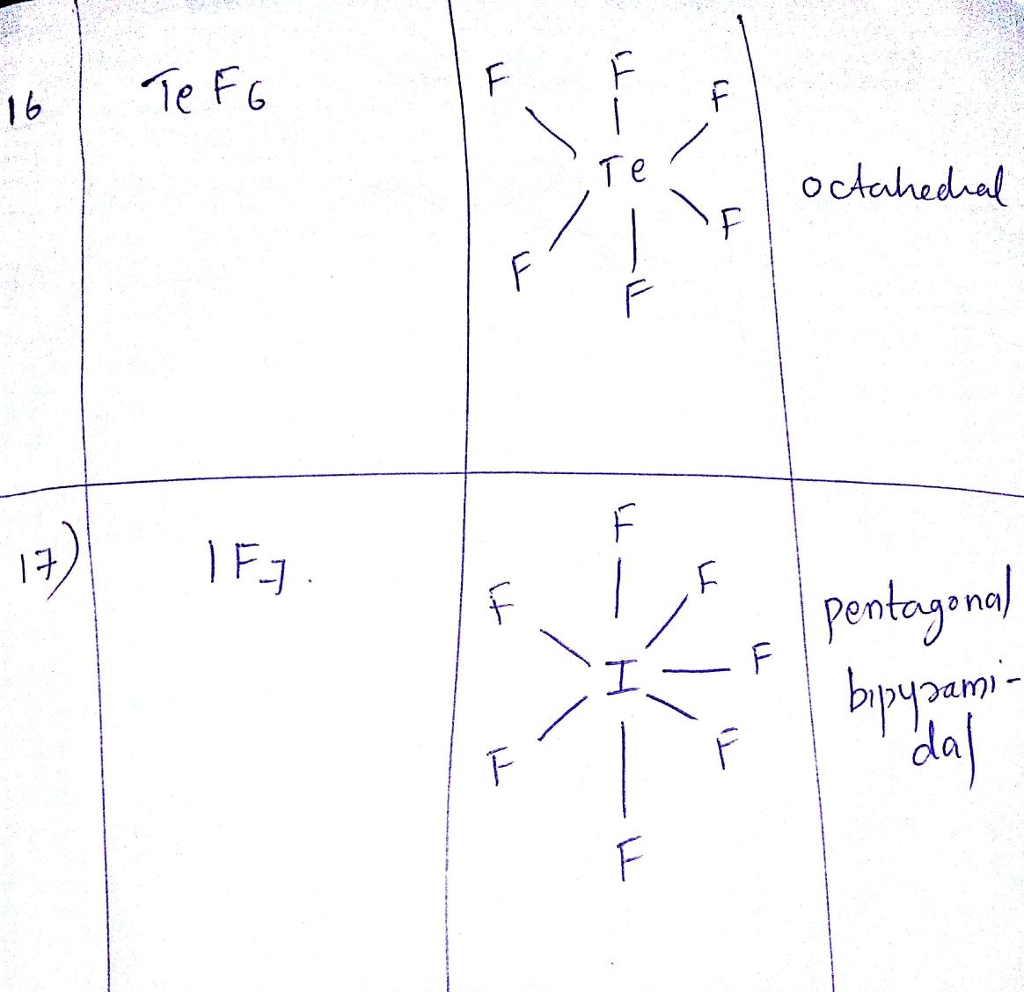#### Earn Coins

Coins can be redeemed for fabulous gifts.

Similar Homework Help Questions
• ### Provide the correct molecular geometry for (c), given the number of lone pairs and bonding groups...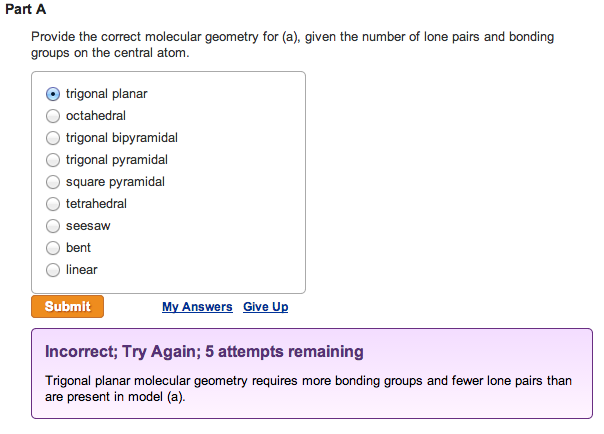Provide the correct molecular geometry for (a), given the number of lone pairs and bonding groups on the central atom. trigonal planar  octahedral  trigonal bipyramidal  trigonal pyramidal  square pyramidal  tetrahedral  seesaw  bent  linearProvide the correct molecular geometry for (b), given the number of lone pairs and bonding groups on the central atom.  trigonal bipyramidal  square pyramidal  bent  tetrahedral  trigonal planar  seesaw  trigonal pyramidal  octahedral  linear Provide the correct molecular geometry for (c), given the number of lone pairs and bonding groups on the...

• ### 10.) Specify the electron-domain geometry for the following two species using the VSEPR model: Xe...

10.) Specify the electron-domain geometry for the following two species using the VSEPR model: XeCl4 and IO4−. For each species, predict the bond angles based on the electron-domain geometry. (a)    XeCl4 geometry: linear trigonal planar    bent tetrahedral trigonal pyramidal trigonal bipyramidal seesaw T-shaped octahedral square pyramidal square planar pentagonal bipyramidal pentagonal pyramidal pentagonal planar bond angle: 60° 72°   90° 109.5° 120° 180° (b)    IO4− geometry: linear trigonal planar    bent tetrahedral trigonal pyramidal trigonal bipyramidal seesaw T-shaped octahedral square pyramidal square...

• ### Specify the electron-domain geometry for the following two species using the VSEPR model: KrCl4 and BrO4....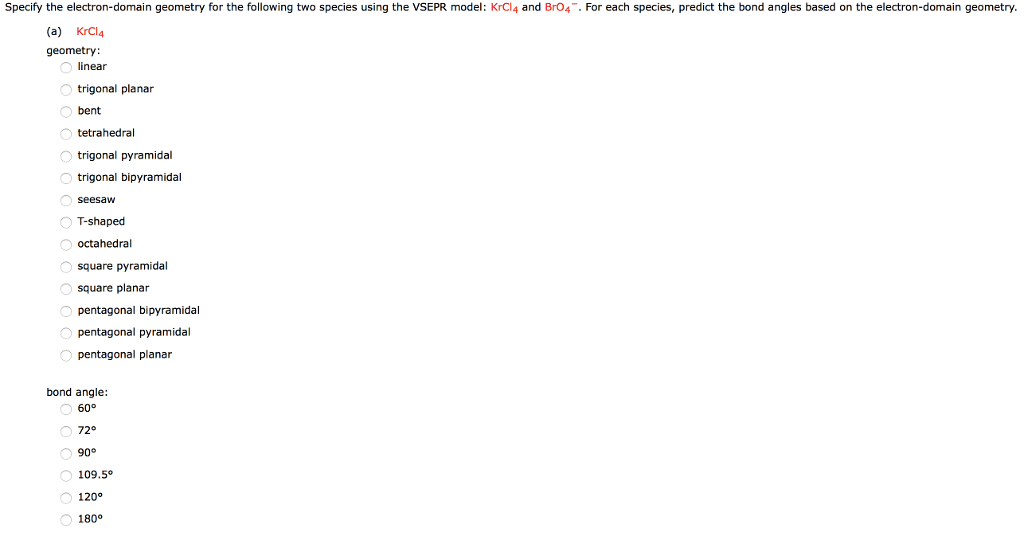Specify the electron-domain geometry for the following two species using the VSEPR model: KrCl4 and BrO4. For each species, predict the bond angles based on the electron-domain geometry. (a) KrCl4 geometry linear trigonal planar bent tetrahedral trigonal pyramidal trigonal bipyramidal T-shaped octahedral square pyramidal square planar pentagonal bipyramidal pentagonal pyramidal pentagonal planar bond angle: 72° 109.5° 120 180° (b) Br04 geometry: inear trigonal planar bent tetrahedral trigonal pyramidal trigonal bipyramidal seesaW T-shaped octahedral square pyramidal square planar pentagonal bipyramidal pentagonal...

• ### VSEPR theory predicts which shape for the molecular structure of the following molecules or molecular ions?...

VSEPR theory predicts which shape for the molecular structure of the following molecules or molecular ions? (i.e. the arrangement of only the bonded atoms about the central atom) bent (or angular) linear octahedral see-saw square planar square pyramidal tetrahedral trigonal bipyramidal trigonal planar trigonal pyramidal T-shaped  ICl3 molecule    (The central atom is I.) bent (or angular) linear octahedral see-saw square planar square pyramidal tetrahedral trigonal bipyramidal trigonal planar trigonal pyramidal T-shaped  IO4- ion    (The central atom is I.) bent (or angular) linear...

• ### Number 1-6 1. What is the molecular geometry of SBr4? A) seesaw B) square planar C)...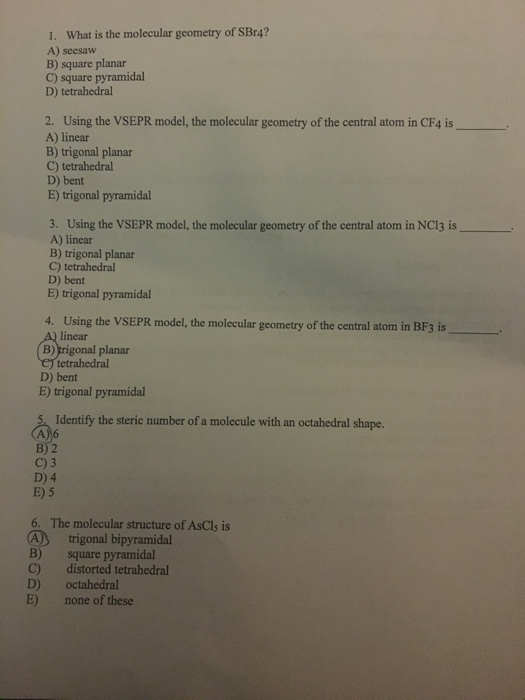Number 1-6 1. What is the molecular geometry of SBr4? A) seesaw B) square planar C) square pyramidal D) tetrahedral 2. Using the VSEPR model, the molecular geometry of the central atom in CF4 is A) linear B) trigonal planar C) tetrahedral D) bent E) trigonal pyramidal 3. Using the VSEPR model, the molecular geometry of the central atom in NC13 is A) linear B) trigonal planar C) tetrahedral D) bent E) trigonal pyramidal 4. Using the VSEPR model, the...

• ### According to VSEPR theory, XeO3 has how many regions of electron density around the central atom...

According to VSEPR theory, XeO3 has how many regions of electron density around the central atom (how many electron groups?) (enter a number from 1 to 6) ? XeO3 will have (linear, trigonal planar, tetrahedral, trigonal pyramidal, bent, trigonal bipyramidal, seesaw, T-shaped, octahedral, square pyramidal, square planar) electronic geometry, and (linear, trigonal planar, tetrahedral, trigonal pyramidal, bent, trigonal bipyramidal, seesaw, T-shaped, octahedral, square pyramidal, square planar) molecular geometry. Draw the best Lewis structure using formal charge information. How many single...

• ### Using VSEPR theory, a molecule with the general formula AX E# (A is the central atom,...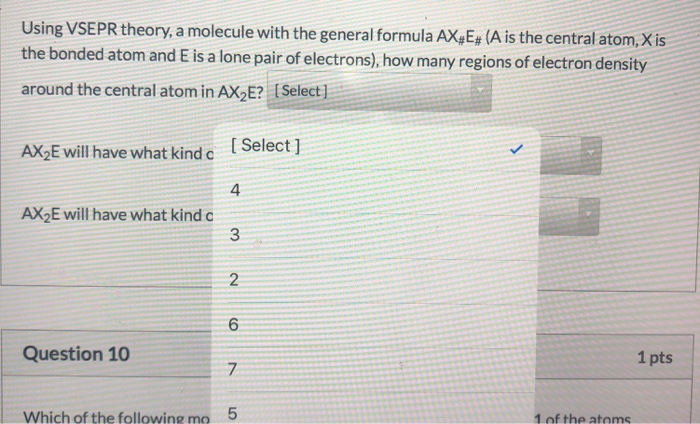Using VSEPR theory, a molecule with the general formula AX E# (A is the central atom, Xis the bonded atom and E is a lone pair of electrons), how many regions of electron density around the central atom in AX E? [Select] AX2E will have what kind c [Select] AX2E will have what kind a w N o Question 10 1 pts V Which of the following mo 5 1 of the atoms 5:35 PM Using VSEPR theory, a molecule...

• ### The arrangement of electron groups around the Xe atom in XeF4 is Group of answer choices...

The arrangement of electron groups around the Xe atom in XeF4 is Group of answer choices tetrahedral. trigonal bipyramidal. square planar. octahedral. square pyramidal.

• ### Which of the following molecular geometries (shapes) are always Polar if 2 of the atoms around...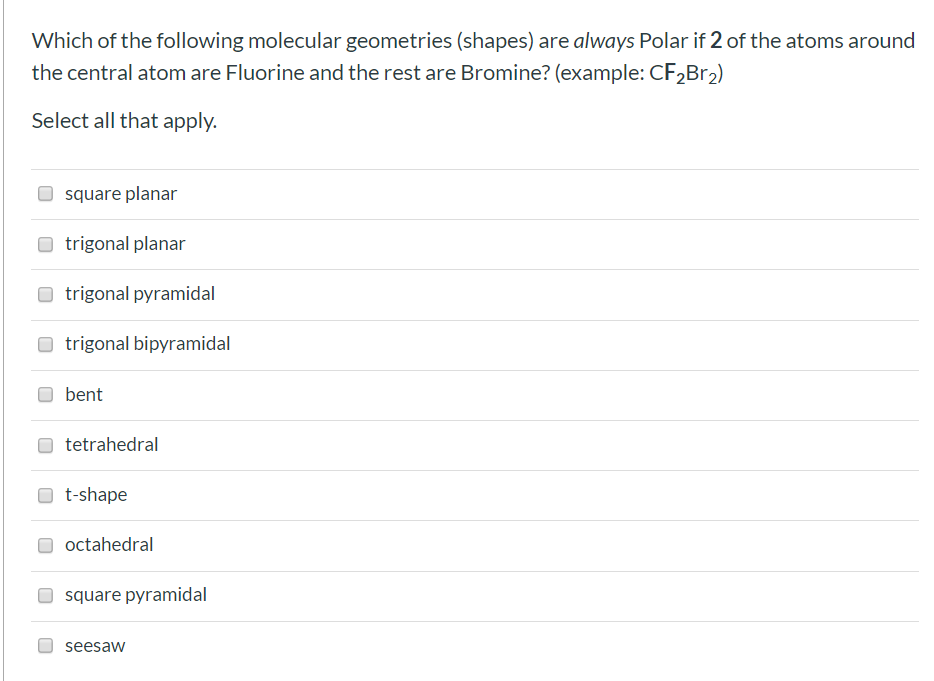Which of the following molecular geometries (shapes) are always Polar if 2 of the atoms around the central atom are Fluorine and the rest are Bromine? (example: CF2Br2) Select all that apply. square planar trigonal planar trigonal pyramidal trigonal bipyramidal bent tetrahedral t-shape octahedral square pyramidal seesaw

• ### Which of the following molecular geometries (shapes) are always Polar if 1 of the atoms around the central atom is...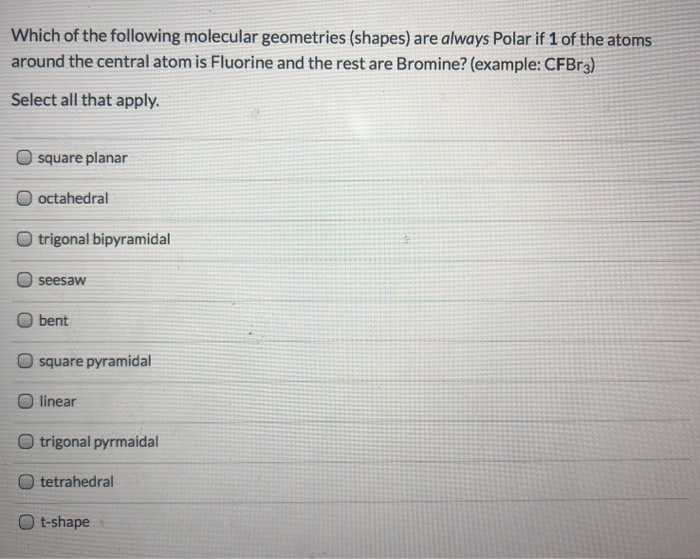Which of the following molecular geometries (shapes) are always Polar if 1 of the atoms around the central atom is Fluorine and the rest are Bromine? (example: CFBr3) Select all that apply. square planar O octahedral trigonal bipyramidal seesaw bent square pyramidal O linear trigonal pyrmaidal tetrahedral t-shape